login  home  contents  what's new  discussion  bug reports help  links  subscribe  changes  refresh  edit

 Submitted by : (unknown) at: 2007-11-17T22:18:55-08:00 (16 years ago) Name : Axiom Version : default friCAS-20090114 Axiom-20050901 OpenAxiom-20091012 OpenAxiom-20110220 OpenAxiom-Release-141 Category : Axiom Aldor Interface Axiom Compiler Axiom Library Axiom Interpreter Axiom Documentation Axiom User Interface building Axiom from source lisp system MathAction Doyen CD Reduce Axiom on Windows Axiom on Linux Severity : critical serious normal minor wishlist Status : open closed rejected not reproducible fix proposed fixed somewhere duplicate need more info Optional subject :   Optional comment :

Originally Savannah bug 10350.

Example of code trigerring the bug:

axiom
integrate((1/x)::UTS(FRAC POLY INT, x,0),x)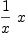(1)
Type: UnivariateTaylorSeries?(Fraction(Polynomial(Integer)),x,0)

axiom
integrate((1/y)::UTS(FRAC POLY INT, x, 0),z)
There are 11 exposed and 8 unexposed library operations named
integrate having 2 argument(s) but none was determined to be
applicable. Use HyperDoc Browse, or issue
)display op integrate
package-calling the operation or using coercions on the arguments
will allow you to apply the operation.
Cannot find a definition or applicable library operation named
integrate with argument type(s)
UnivariateTaylorSeries(Fraction(Polynomial(Integer)),x,0)
Variable(z)
Perhaps you should use "@" to indicate the required return type,
or "$" to specify which version of the function you need. The first bug is an indication that 1/x should not be of type UTS(x, FRAC POLY INT), UP(x, FRAC POLY INT) and so on. The second is an indication that QFCAT should have a function variables: % -> List Symbol. Sat 10/02/04 at 08:09, comment #2: I disagree with the previous comment; x should be captured and not allowed in the FRAC POLY INT. (I agree that the second example in the original report is not a bug.) But surely we can all agree that integrate((1/x)::ULS(FRAC POLY INT, x, 0),x) is a bug? The problem is the same: the 1/x is being treated in the coefficient domain. Note that integrate(1/(x::ULS(FRAC POLY INT, x, 0)),x) produces a correct result. Dylan (dpt@math.harvard.edu) Sat 10/02/04 at 04:33, comment #1: Neither of these is a bug. In the first one, Axiom coerced 1/x into FRAC POLY INT correctly: the only / operation available in UTS is one induced from the coefficient domain, which requires the denominator to be in the coefficient domain, and the division is done termwise to the coefficients of the series. So 1/x ends up in FRAC POLY INT. Note that to obtain a Taylor series at x = 0 is mathematically is wrong, since 1/x is not defined at x=0. Also the way to obtain a Taylor series is taylor(func, x=a). If you do integrate(taylor(1/x,x=1),x), that would cause no problems. Note that the domain of this command is UTS(EXPR INT, x,1), so such towers are valid and necessary in Axiom. Note also there are only two 'exported[coerce]?' in UTS and they do NOT apply to 1/x. The x in UTS is like the x in UP and is different from the x in FRAC POLY INT. The representation is Stream Coef (no variable specified because it is univariate). For the same reason, in the second command, 1/y is correctly coerced into FRAC POLY INT. However, in UTS(*,x,*), the only integrations allowed are with respect to x. If you want to do integration in FRAC POLY INT, then you should do so without coercing 1/y into UTS. So your examples do not illustrate the problem about mixed up variables. In fact, it supports use of towers like UTS(EXPR INT, x, a). William (wyscc@cunyvm.cuny.edu) not a bug --Bill Page, Sat, 18 Feb 2006 11:10:37 -0600 reply The result is a series: axiom integrate((1/x)::ULS(FRAC POLY INT, x, 0),x)(2) Type: UnivariateLaurentSeries?(Fraction(Polynomial(Integer)),x,0) It is finite so we can represent it as a polynomial: axiom univariatePolynomial(%,1)(3) Type: UnivariatePolynomial(x,Fraction(Polynomial(Integer))) This domain distinquishes between x as a monomial and 1/x as a coefficient, but in a simpler domain they will cancel: axiom %::FRAC POLY INT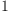(4) Type: Fraction(Polynomial(Integer)) 1/x x = 1 is a bug! --wyscc, Thu, 23 Feb 2006 01:07:56 -0600 reply I think recently this issue of using the same identifier x to stand for two different variables in two different domains (either accidentally or on purpose) has been thoroughly discussed, but not quite. Note that I distinguish identifier from variable. We have two variables, usually one internally denoted by the Symbol x and another unspecified as ? (as in UP(x,INT)) where ? does NOT exist but can either be printed as ? or any identifier the user prefers, such as x. As any one who does programming should know, the same identifier should never be used to denote two different objects (at least not within the same scope). By the way, this design is in some sense ingenious, and necessary, to allow extending a polynomial ring that already has all the symbols as variables; such extension is useful in testing radical ideal membership of polynomial rings, and intersections of polynomial ideals, for example. In creating integrate((1/x)::ULS(FRAC POLY INT, x, 0),x), the user erred by combining two operations into one and thus allowing, actually forcing, Axiom's Interpreter to firstly misidentify the first x encountered during scan as belonging to FRAC POLY INT when the user meant it to be in ULS(FRAC POLY INT, x, 0) and then secondly it is forced to coerce the result 1/x to be a coefficient in ULS(FRAC POLY INT,x,0) by the user. Once satisfied that the result is in ULS(FRAC POLY INT, x, 0), there is no need to do anything further but to start processing the integrate command. Instead, the user should have separated the two operations: axiom f:ULS(FRAC POLY INT, x,0):=1/x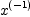(5) Type: UnivariateLaurentSeries?(Fraction(Polynomial(Integer)),x,0) axiom integrate(f,x) >> Error detected within library code: integrate: series has term of order -1 Three things should be noted here: (a) x is first encountered in ULS(FRAC POLY INT, x,0) which creates an identifier x for the main variable ? in the ULS domain. (b) In the first line, having bound x to the main variable, 1/x is now treated as a Laurent series (not as a coefficient living in FRAC POLY INT), but it is not apparent to the inexperienced user in the output (but it is different, as seen below). (c) In the second line, Axiom fails (correctly) because the integral, which is log(x), which is not given by a Laurent series about x=0. A better example is: axiom g:ULS(FRAC POLY INT, x,0):=1/(x-1)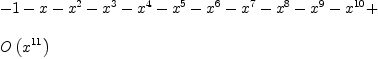(6) Type: UnivariateLaurentSeries?(Fraction(Polynomial(Integer)),x,0) axiom integrate(g,x)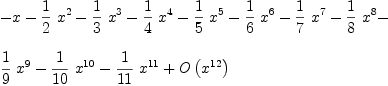(7) Type: UnivariateLaurentSeries?(Fraction(Polynomial(Integer)),x,0) Here Axiom actually expands 1/(x-1) in a series in powers of x. Compared this with: axiom h:=(1/(x-1))::ULS(FRAC POLY INT, x,0)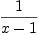(8) Type: UnivariateLaurentSeries?(Fraction(Polynomial(Integer)),x,0) axiom integrate(h,x)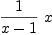(9) Type: UnivariateLaurentSeries?(Fraction(Polynomial(Integer)),x,0) Now Axiom having been forced to output the same identifier for two different variables, the first x bound to a variable in FRAC POLY INT, and the second x bound to the main variable of ULS, should NOT then simplify the expression 1/x x of (3) to 1. This is unfortunately a consequence of the design goal to permit elements of UP(x,R) to be coercible to POLY R where the main variable is coerced into the variable using the identifier x. In our case, ULS(R,x,0) was first truncated to UP(x,R) (using univariatePolynoimial) by identifying the main variable of ULS with the main variable of UP(x,R). Here R IS FRAC POLY INT, and UP(x, FRAC POLY INT) is first coerced into FRAC UP(x, POLY INT) and then further to FRAC POLY INT via UP(x, POLY INT) to POLY INT (from map from FRAC2(UP(x, POLY INT),POLY INT) and the Interpreter probably simplified POLY POLY INT to POLY INT). In other words, we have ((1/x ?)::(?/x)::(x/x)=1. The bug therefore probably lies in the identification of POLY POLY INT with POLY INT where the outer ? (or x) is identified with x of the inner POLY. Conclusion: (i) Any simplification of towers which makes sense in mathematics probably would not make sense in computer algebra (due to scope problems for one). Other examples could be FRAC FRAC INT to FRAC INT and EXPR EXPR INT to EXPR INT. (ii) Users should be alert to the order in which an input is scanned and the consequences, and break a long input into simpler steps. (iii) Users should not use the same identifier for two different objects. William Users should not use the same identifier for two different objects. consider that this is COMPUTATIONAL mathematics. the user has to know what the scope of a variable is. the bug is actually a user error. the behavior of the system is well defined and the result can be predicted. failure to correctly scope variables is a standard programmer error. removing simplification of type towers would significantly reduce the usefulness of axiom. t 1/x x = 1 is a feature(?) --wyscc, Sat, 25 Feb 2006 16:56:22 -0600 reply Now Axiom having been forced to output the same identifier for two different variables, the first x bound to a variable in FRAC POLY INT, and the second x bound to the main variable of ULS, should NOT then simplify the expression 1/x x of (3) to 1. I have examined this simplification more closely. As discussed in another email, this is actually done by invoking a substitution map: briefly, the x in ULS is being substituted by the x in FRAC POLY INT. I think this is unwarranted "smartness" by the Interpreter (this is a "lesser crime" than a "bug"). Of course, as Tim said above, this is secondary error due to an error by the user. It would be a legitimate substitution if a user initiated it. I believe the automatic substitution would not be done in the compiler (that is, the coercion would be flagged). The Axiom compiler, as far as I can tell, scoped the two x's correctly. It is the automatic substitution by the Interpreter due to a request to coerce that yields the answer 1. Whether you call this a feature or a bug is up to you. Users beware. William From email referred to above: (edited for Wiki) :: Date: Fri, 24 Feb 2006 07:14:24 -0500 From: William Sit To: bill.page1@synthesis.anikast.ca Bill:  > This thread seems to go on forever ... but I feel compelled > to continue it because: 1) I think understanding how this > works is critical to understanding and using Axiom, 2) I don't > think we have a simple explanation yet of something that really > should be simple in principle, if not in it's application in > certain cases.  There never is anything simple in Axiom. By its nature every function is parametrized and categorical. Clearly, this issue will recur as new users stumbles onto these constructions. As you know, a polynomial ring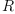over a ring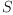in the indeterminates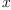1, ...,n, means that1, ...,n are algebraically independent over; in particular, they are not elements of. If you really want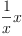to mean 1, the two's must live in the same domain. Either you put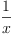in the coefficient domainand then err on using the same identifier for a transcendental over, or you should simply use thefromin formingin the quotient field of(assumingis an integral domain). When you write UP(x, FRAC POLY INT), you specifically told Axiom that x (more precisely, the indeterminate ? with an identifier x) is transcendental over FRAC POLY INT. So you should not use x in FRAC POLY INT. The fact that Axiom cannot disallow you to do that is not a license to violate this mathematical definition! In any computer language, there are always unforseen constructions that a compiler cannot diagnose and sometimes, such abuse may be used for tricky programming, but they are abuse nonetheless. For old FORTRAN programmers, this is similar to core to core I/O where you can turn an integer into a real with the same binary representation. FORTRAN allows this, but you can't possibly say mathematically the two are the same! When a programmer deliberately violates the rules of construction that cannot be caught by the compiler, it is a bug of the compiler. However, no matter how buggy a computer algebra system is, we are using it to do mathematics and so no mathematical rules should be violated in the computations. As to your quibble to my separating "identifier", let me emphasize: identifiers are external strings what a user in the interpreter use to refer to objects that are internal to Axiom. Symbol and Variable are domains in Axiom and their objects are internal. The fact that we use the same identifier string as the representation string for a Symbol object is only a convenience. As you pointed out, a Symbol object need not be a simple string and can be adorned with all sorts of scripts. You would not want to keep inputting a symbol adorned in a complicated way in its full detail (note the linear form to enter such a symbol is a function, not the representation). So if I say axiom )set mess bot on y:=x[1,2,3,4] Function Selection for x Arguments: LIST(PI) -> no appropriate x found in List(PositiveInteger) -> no appropriate x found in List(Integer) -> no appropriate x found in PositiveInteger -> no appropriate x found in List(PositiveInteger) -> no appropriate x found in List(Integer) -> no appropriate x found in PositiveInteger -> no function x found for arguments LIST(PI) Function Selection for elt Arguments: (VARIABLE(x),LIST(PI)) -> no appropriate elt found in Variable(x) -> no appropriate elt found in List(PositiveInteger) -> no appropriate elt found in Symbol -> no appropriate elt found in List(Integer) -> no appropriate elt found in PositiveInteger -> no appropriate elt found in Variable(x) -> no appropriate elt found in List(PositiveInteger) -> no appropriate elt found in List(Integer) -> no appropriate elt found in PositiveInteger  signature: (SYMBOL,LIST(OUTFORM)) -> SYMBOL implemented: slot $$(List (OutputForm)) from SYMBOL Function Selection for elt Arguments: (VARIABLE(x),LIST(PI))  signature: (SYMBOL,LIST(OUTFORM)) -> SYMBOL implemented: slot$$(List (OutputForm)) from SYMBOL Function Selection for map by coercion facility (map) Arguments: ((PI -> OUTFORM),LIST(PI)) Target type: LIST(OUTFORM) -> no appropriate map found in List(PositiveInteger) -> no appropriate map found in List(OutputForm) -> no appropriate map found in OutputForm -> no appropriate map found in PositiveInteger -> no appropriate map found in PositiveInteger -> no appropriate map found in OutputForm Modemaps from Associated Packages  ((D4 -> D5),List(D4)) -> List(D5) from ListFunctions2(D4,D5) if D4 has TYPE and D5 has TYPE  signature: ((PI -> OUTFORM),LIST(PI)) -> LIST(OUTFORM) implemented: slot (List (OutputForm))(Mapping (OutputForm) (PositiveInteger))(List (PositiveInteger)) from LIST2(PI,OUTFORM)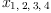(10) Type: Symbol then:  y is the identifier I use (y is NOT the symbol), x[1,2,3,4] the linear function with signature elt: (VARIABLE x, LIST PI)->SYMBOL, and the symbol is x with [1,2,3,4] as subscript x itself is a Symbol and is different (as far as Axiom goes) from the x in the domain Variable(x)  Just to emphasize the fine distinctions, the output you see, is an element of OUTPUTFORM! (another string representing some internal object of the domain Symbol). Bill Page wrote:  > William Sit continued: > > > This is unfortunately a consequence of the design goal to > > permit elements of UP(x,R) to be coercible to POLY R where > > the main variable is coerced into the variable using the > > identifier x. ... > > I don't think that this is the case since we can obtain the > same result above using: > > (1/x)$MPOLY([x],FRAC POLY INT)*x
>   %::FRAC MPOLY([x],POLY INT)


I am trying to explain the coercion using UP(x,R) as a generic representative. Sure, you can violate the rule of construction using any of the polynomial domains in Axiom. The short answer is, any indeterminate you use in the construction of a polynomial domain is transcendental over the coefficient domain and should NOT be coercible to the coefficient domain! I am saying, the above design goal is flawed in illegal constructions like these, but makes sense in the legal construction:

  coerce: UP(x,R) -> POLY R


when R does not contain x (and it shouldn't contain x, and if it does, wrongly, in concept, then x should not be instantiated there).

  > > Here R IS FRAC POLY INT, and UP(x, FRAC POLY INT) is first
> > coerced into FRAC UP(x, POLY INT) and then further to
> > FRAC POLY INT via UP(x, POLY INT) to POLY INT (from map
> > from FRAC2(UP(x, POLY INT),POLY INT)
>
> Apparently on the first coercion is sufficient. Moving the
> fraction from the coefficient domain to become a fraction
> of polynomials:
>
>   UP(x, FRAC POLY INT) +-> FRAC UP(x, POLY INT)
>
> provides a domain in which the two uses of x can cancel.

You are missing the point.

I don't care how the Interpreter performs the illegal coercion (except that I was trying to explain the design goal that leads to the illegal coercion). The coercion is simply WRONG. Period. It is an error built on a user error. (Don't we know that compilers are famous for that when reporting error messages?) In such cases, a user is supposed to remove the original error, not argue about the validity or invalidity of subsequent errors.

  > In fact we can see this same coercion in operation in the
> case of going from:
>
>   POLY FRAC INT +-> FRAC POLY INT
>
> (1) -> (1/2*x)$UP(x, FRAC INT) > > 1 > (1) - x > 2 > Type: UnivariatePolynomial(x,Fraction Integer) > > (2) -> )set message bottom on > (2) -> %::FRAC UP(x,INT) [additonal output snipped]  These are legal coercions since mathematically, POLY FRAC INT is a subring of FRAC POLY INT and similarly, UP(x, FRAC INT) is a subring of FRAC UP(x, INT). The design goal I mentioned above is not involved. If you had taken x/2 in FRAC POLY INT or in POLY FRAC INT and coerce this to UP(x, FRAC INT), then the design goal is applied and the Interpreter (not the compiler) will oblige by identifying the the variable x of POLY FRAC INT with the main variable ? of UP(x, FRAC INT) because the user used the identifier x for ?. This feat is accomplished only with the help of UP2(x,POLY FRAC INT,x,FRAC INT), which explicitly maps the x in POLY FRAC INT to the x (or ?) of UP(x, FRAC INT). It is equivalent to a substitution map. There is no general coercion from POLY FRAC INT to UP(x, FRAC INT).  > > Conclusion: (i) Any simplification of towers which makes > > sense in mathematics probably would not make sense in > > computer algebra (due to scope problems for one). Other > > examples could be FRAC FRAC INT to FRAC INT and EXPR EXPR > > INT to EXPR INT. > > I don't understand this comment. What do you mean by > "scope problems"?  When one constructs POLY POLY INT, one tells Axiom to use Symbol as the variable set over POLY INT. Thus the Symbol in the outer construction should be in a different lexicon scope so that this copy of Symbol can be a family of algebraically independent elements over POLY INT. I don't want to go into the details of how domains are constructed (I probably don't know anyway), but if we modify whatever will be used as symbols in POLY INT by attaching a context prefix, say POLYINT-x for what we normally use x, then we can distinguish another symbol x in this copy for the external POLY construction by using POLYPOLYINT-x. Apparently, the Interpreter allows a substitution of POLYPOLYINT-x by POLYINT-x, giving the user the impression that the two are identified, and this is the flaw I was discussing. The Interpreter should not do that with coercion. A substitution should be explicitly requested by the user. A coercion should not involve a hidden substitution. Let me repeat this mathematically. Letbe a ring,a set and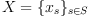be a family of algebraic indeterminates over. Then we can construct the polynomial ring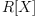. If we like, we can let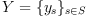be another family of algebraic indeterminates overand construct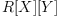. We can make substitution maps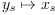, but we should NEVER identify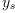with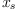and say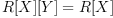simply because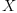and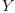are indexed by the same set. No one would argue that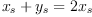is correct, or the derivative of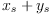with respect tois 2 and not 1. You can substituteby Integer andby Symbol. The problem with the computer algebra case is that Symbol is like the set of all symbols in concept, and a priori prevents construction of any other symbol outside Symbol. This is a very bad restriction. We want to be able to talk about unknowns over EXPR INT, say in differential equations. In reality, only finitely many symbols are instantiated and hence we can solve the problem using context-prefix (scope separation). So while this all encompassing concept may be convenient, it should be taken with a grain of salt. Mathematica for example uses context-prefixes in their packages. The construction of FRAC FRAC INT is similar, except that in this case, there is no argument that ifis an integral domain, and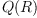its quotient field, then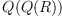is isomorphic to. But it is precisely that the setsandare not the same set, but just isomorphic (even canonically), that requires the implelmentation of the isomorphism in computer algebra. One simply cannot "identify" the two sets in computer algebra because they do have different data representations. In order to implement the isomorphism, one must be able to distinguish when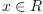is regarded as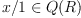or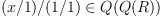. One way would be to use context-prefix. To the naive users, this seems really much ado about nothing: Axiom relies on users not to construct (or explicitly disallows in the Interpreter) such towers. However, this is only ok up to a point. When one can write routines that return domains, this naive design forces a case by case examination of the returned domains to screen out "trivial" (I would prefer the adjective "boundary") cases (for example, if I want to construct FRAC R where R is a run-time computed domain, then I need to use something like if R has FIELD then R in FRAC R construction). Unfortunately, the pedantic (isomorphism) way is very inefficient so a compromise was taken. A big big question in computer algebra should be how to handle "canonically isomorphic" mathematical objects. Mathematicians assume these identifications without a thought in most cases, but there are occasions where distinction is important. For example, they would rarely identify two vastly differently defined finite sets just because they have the same cardinality.  > > (ii) Users should be alert to the order in which an input is > > scanned and the consequences, and break a long input into > > simpler steps. > > I agree that simple steps are easier to analyze. I also > think it is a mistake to write something like: > > (x+1)::POLY INT > > or > > x+1::POLY INT > > when > > (x+1)$POLY INT
>
> will do. In the first two cases the interpreter will do
> some extra computation by default before it applies the
> coercion operation and the result of coercion is harder
> to analyze than the simple package call.
Agreed.
>  (iii) Users should not use the same identifier for two
> > different objects.
>
> I think that this is simply not possible in Axiom.

I am not talking about spad code, where there are multiple scopes. In the
interpreter, unless you use a different frame, all identifiers the user uses are
in one scope. If you use x for UP(x, POLY INT), then you should not use x in
POLY INT. May be you can give me an example where you MUST use (or at least it
is desirable to use) the same identifier for two different objects in an
Interpreter session?
William

The following is verbatim quote from Email by Bill Page:
-------- Original Message --------
Subject: Re: 1/x x = 1 is a bug!
Date: Fri, 24 Feb 2006 00:58:19 -0500
From: "Page, Bill"
To: "William Sit"
CC: , ,"Martin Rubey" ,"Ralf Hemmecke" ,
William,
This thread seems to go on forever ... but I feel compelled
to continue it because: 1) I think understanding how this
works is critical to understanding and using Axiom, 2) I don't
think we have a simple explanation yet of something that really
should be simple in principle, if not in it's application in
certain cases.
As a long term user of Axiom, I really appreciate you input
on this subject. But I think I must take exception to your
conclusion as stated in the subject line. :)
On Thu, 23 Feb 2006 01:07:56 -0600 at
http://wiki.axiom-developer.org/270IntegratingUTS#bottom
You wrote:

Now Axiom having been forced to output the same identifier
for two different variables, the first x bound to a variable
in FRAC POLY INT, and the second x bound to the main variable
of ULS, should NOT then simplify the expression 1/x x of (3)
to 1.
First, I would prefer that we try not to introduce new words
like "identifier" since Axiom already calls these by the name
Symbol. Second, I worry that you are using the word "bound"
in a manner that does not really apply to Axiom. And third,
I think the use of the word "variable" in Axiom has to be
applied with some care.
When you say "x bound to a variable in FRAC POLY INT" I
think you mean only that the symbol x is used as a
polynomial variable in this domain. Exactly what a
"polynomial variable" is, is defined by the specific
implementation of FRAC POLY INT, but we know for sure
that it will appear in the List of Symbols that is returned
by the function variables when applied to any member of
this domain. And these symbols also play a role in many
other functions exported by this domain.
If I write things below that are completely obvious to you,
please bear with me and consider it just a short review. Of
course also correct me if I get it wrong. I am assuming that
there are other readers who need might this sort of review.
As you know, Symbols themselves form a domain in Axiom:
)sh Symbol
I think there is a lot of unnecessary confusion between
symbols and variables.
By definition Symbols are constants and can not be assigned
values. Operations like  = : (Symbol,Symbol) -> Boolean

compare one symbol to another. There are other operations that
construct new symbols possibly with sub/super-scripts etc. It
might be surprising how sophisticated Axiom's Symbol domain is
and how much of it is coded in SPAD:
But of course ultimately all Symbols are represented and
manipulated by Lisp, e.g.:    coerce(s:String):%     == VALUES(INTERN(s)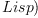Lisp
x = y                  == EQUAL(x,y)$Lisp Variables also form a domain in Axiom: )sh Variable Variables are constructed from Symbols, but not all symbols are necessarily used for this purpose. Members of the Variable domain in turn can be assigned values and have associated types. It may seem strange but Variables are not used to form polynomials in Axiom. ---------- Let's consider the following somewhat simplified example where Axiom will coerce 1/x x to 1: (1) -> (1/x)$UP(x,FRAC POLY INT)*x

(1)  - x
x
Type: UnivariatePolynomial(x,Fraction Polynomial Integer)

Here 1/x is a coefficient and polynomial variable x is
written to the right of the coefficient. Both uses of x
represent the same Symbol and neither of these are from the
domain Variable.
There is no operation within the domain UP(x,FRAC POLY INT)
which would allow us to conclude that 1/x x is identical
to 1 even though these are both members of this domain. We
know intuitively however that this expression should be 1
in some other domain, but showing this formally apparently
involves some rather deep knowledge about "fractional ideals"
that is beyond me.
(2) -> )set message bottomup on
(2) -> )set message autoload on
(2) -> %::FRAC UP(x,POLY INT) Function Selection for map by coercion facility (map)
Arguments: ((POLY INT -> UP(x,POLY INT)),FRAC POLY INT)
Target type: FRAC UP(x,POLY INT)
-> no appropriate map found in Fraction Polynomial Integer
-> no appropriate map found in
Fraction UnivariatePolynomial(x,Polynomial Integer)
-> no appropriate map found in
UnivariatePolynomial(x,Polynomial Integer)
-> no appropriate map found in Polynomial Integer
-> no appropriate map found in
UnivariatePolynomial(x,Polynomial Integer)
Modemaps from Associated Packages
 ((D7 -> D11),FractionalIdeal(D7,D8,D9,D10)) ->
FractionalIdeal(D11,D1,D2,D3)
from FractionalIdealFunctions2(D7,D8,D9,D10,D11,D1,D2,D3)
if D7 has EUCDOM and D8 has QFCAT D7 and D9 has UPOLYC D8
and D10 has Join(FramedAlgebra(D8,D9),RetractableTo D8) and
D11 has EUCDOM and D1 has QFCAT D11 and D2 has UPOLYC D1
and D3 has Join(FramedAlgebra(D1,D2),RetractableTo D1)
 ((D4 -> D5),Fraction D4) ->
Fraction D5 from FractionFunctions2(D4,D5)
if D4 has INTDOM and D5 has INTDOM
  signature:   ((POLY INT -> UP(x,POLY INT)),FRAC POLY INT) ->
FRAC UP(x,POLY INT)
implemented: slot (Fraction (UnivariatePolynomial x (Polynomial
(Integer))
))(Mapping (UnivariatePolynomial x (Polynomial (Integer))) (Polynomial
(Integer)
package FractionFunctions2
package QuotientFieldCategoryFunctions2
package PolynomialGcdPackage
(2)  1
Type: Fraction UnivariatePolynomial(x,Polynomial Integer)

-----------
William Sit continued:

This is unfortunately a consequence of the design goal to
permit elements of UP(x,R) to be coercible to POLY R where
the main variable is coerced into the variable using the
identifier x. ...
I don't think that this is the case since we can obtain the
same result above using:  (1/x)$MPOLY([x],FRAC POLY INT)*x %::FRAC MPOLY([x],POLY INT) Here R IS FRAC POLY INT, and UP(x, FRAC POLY INT) is first coerced into FRAC UP(x, POLY INT) and then further to FRAC POLY INT via UP(x, POLY INT) to POLY INT (from map from FRAC2(UP(x, POLY INT),POLY INT) Apparently on the first coercion is sufficient. Moving the fraction from the coefficient domain to become a fraction of polynomials: UP(x, FRAC POLY INT) +-> FRAC UP(x, POLY INT) provides a domain in which the two uses of x can cancel. In fact we can see this same coercion in operation in the case of going from: POLY FRAC INT +-> FRAC POLY INT (1) -> (1/2*x)$UP(x, FRAC INT)

(1)  - x
2
Type: UnivariatePolynomial(x,Fraction Integer)

(2) -> )set message bottom on
(2) -> %::FRAC UP(x,INT) Function Selection for map by coercion facility (map)
Arguments: ((INT -> UP(x,INT)),FRAC INT)
Target type: FRAC UP(x,INT)
-> no appropriate map found in Fraction Integer
-> no appropriate map found in
Fraction UnivariatePolynomial(x,Integer)
-> no appropriate map found in
UnivariatePolynomial(x,Integer)
-> no appropriate map found in Integer
-> no appropriate map found in
UnivariatePolynomial(x,Integer)
Modemaps from Associated Packages
 ((D7 -> D11),FractionalIdeal(D7,D8,D9,D10)) ->
FractionalIdeal(D11,D1,D2,D3)
from FractionalIdealFunctions2(D7,D8,D9,D10,D11,D1,D2,D3)
if D7 has EUCDOM and D8 has QFCAT D7 and D9 has UPOLYC D8
and D10 has Join(FramedAlgebra(D8,D9),RetractableTo D8) and
D11 has EUCDOM and D1 has QFCAT D11 and D2 has UPOLYC D1
and D3 has Join(FramedAlgebra(D1,D2),RetractableTo D1)
 ((D4 -> D5),Fraction D4) -> Fraction D5 from
FractionFunctions2(D4,D5)
if D4 has INTDOM and D5 has INTDOM
  signature:   ((INT -> UP(x,INT)),FRAC INT) -> FRAC UP(x,INT)
implemented: slot (Fraction (UnivariatePolynomial x
(Integer)))(Mapping (U
nivariatePolynomial x (Integer)) (Integer))(Fraction (Integer)) from
FRAC2(INT,U
package FractionFunctions2
package QuotientFieldCategoryFunctions2
package HeuGcd
package InnerModularGcd
EuclideanModularRing
package ModularDistinctDegreeFactorizer
domain ModularRing        x
(2)  -
2
Type: Fraction UnivariatePolynomial(x,Integer)

and the Interpreter probably simplified POLY POLY INT to
POLY INT). In other words, we have ((1/x ?)::(?/x)::(x/x)=1.
The bug therefore probably lies in the identification of
POLY POLY INT with POLY INT where the outer ? (or x) is
identified with x of the inner POLY.

I don't see this happening anywhere above. On the contrary this
calculation seems to me to demonstrate the extraordinary power
of the generic algorithms that are part of the Axiom library.

Conclusion: (i) Any simplification of towers which makes
sense in mathematics probably would not make sense in
computer algebra (due to scope problems for one). Other
examples could be FRAC FRAC INT to FRAC INT and EXPR EXPR
INT to EXPR INT.
I don't understand this comment. What do you mean by
"scope problems"?

(ii) Users should be alert to the order in which an input is
scanned and the consequences, and break a long input into
simpler steps.
I agree that simple steps are easier to analyze. I also
think it is a mistake to write something like:  (x+1)::POLY INT

or
x+1::POLY INT
when
(x+1)\$POLY INT
will do. In the first two cases the interpreter will do
some extra computation by default before it applies the
coercion operation and the result of coercion is harder
to analyze than the simple package call.

(iii) Users should not use the same identifier for two
different objects.
I think that this is simply not possible in Axiom.
Regards,
Bill Page.



 Subject:   Be Bold !! ( 14 subscribers )